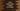# Kotlin program to calculate the total number of digits in a number### Introduction :

In this Kotlin programming tutorial, we will learn how to calculate the total number of digits in a number. For example, 1245 has four digits. This example is mainly for Kotlin beginners. If you have started learning Kotlin after Java, we have also included the Java version of the same problem. It will show you :

1. How to read a number in Kotlin using Scanner class.
2. How to print a string and number in Kotlin
3. How to use while loop in Kotlin.

Let’s take a look at the program :

### Kotlin Program :

``````import java.util.*

fun main(args: Array) {
//1
val scanner = Scanner(System.`in`)

//2
println("Enter a number : ")
var number: Int = scanner.nextInt()

//3
var count = 0
//4
while (number > 0) {
count++
number /= 10
}

//5
println("Total number of digits : \$count")
}
``````

### Explanation :

_The commented numbers in the above program denote the step number below : _

1. Create one Scanner object to read the user input values.
2. Ask the user to enter a number. Read it using the Scanner variable we have created above and store it in an integer variable number.
3. Create one variable count with value 0. This variable will hold the count of digits in the given number.
4. Run one while loop till the value of input number is more than zero. Inside the loop, increment the value of count and change the value of the number to number/10.
5. Finally, print out the result to the user.

### Sample Output :

``````Enter a number :
12345
Total number of digits 5

Enter a number :
1
Total number of digits : 1

Enter a number :
12
Total number of digits : 2
``````

### Java version :

Let’s take a look at the Java version :

``````import java.util.Scanner;

class Main {
public static void main(String[] args) {
Scanner scanner = new Scanner(System.in);
System.out.println("Enter a number : ");

int number = scanner.nextInt();
int count = 0;

while (number > 0) {
count++;
number /= 10;
}

System.out.println("Total number of digits " + count);
}
}
``````

The output will be the same as the previous one.

### Conclusion :

We have learnt how to use while loop to calculate the total number of digits in a number in Kotlin. We have also compared the same program with its Java version. Try to run it on your system and let me know if anything is missed or if anything needs to be added.